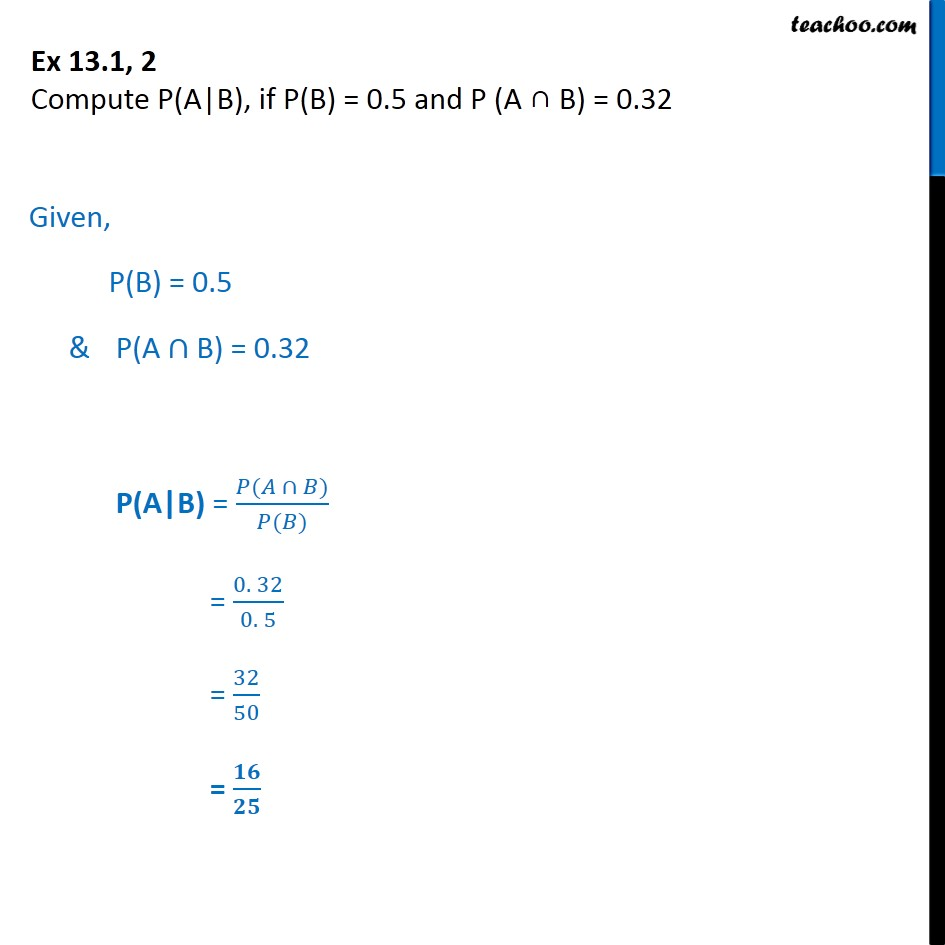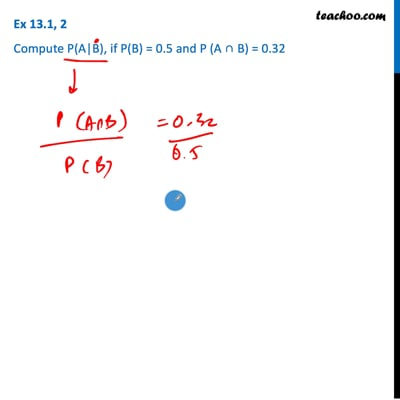Conditional Probability - Values given

Chapter 13 Class 12 Probability
Concept wiseThis video is only available for Teachoo black users

Introducing your new favourite teacher - Teachoo Black, at only ₹83 per month

### Transcript

Ex 13.1, 2 Compute P(A|B), if P(B) = 0.5 and P (A B) = 0.32 Given, P(B) = 0.5 & P(A B) = 0.32 P(A|B) = ( ) ( ) = 0. 32 0. 5 = 32 50 =